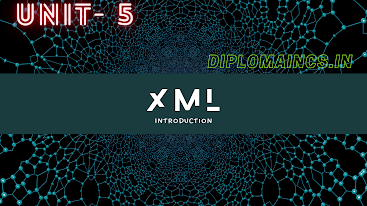## 3/22/21

### Data structure using C diploma question

This question was conducted only for LE (Letral Entry) students in the month of march -2021

(Regular student's exam was postpone due to COVID-19)

Sub Code: - 1618401

Special Exam

2020(Even)

Time : 3Hrs

Semester  IV(New)/CSE

Data Structure Using ‘C’

Full Marks : 70

Pass marks : 28

Group A

Choose the most suitable answer from the following options: -   (1*20=20)

(i) Preorder is as same as:

(a) Depth-first order

(c)  Topological order

(d) Liner order

(ii) Which of the following traversal techniques lists the nodes of binary search tree in ascending order?

(a) Post-order

(b) In-order

(c) Preorder

(d) None of the above

(iii) Merge short uses

(a) Divide and conquer strategy

(b) Backtracking approach

(c) Heuristic search

(d) Greedy approach

(iv) The post fix form A + (B*C) is

(a) ABC+*

(b)ABC*+

(c) AB+C*

(d) AB*C+

(v) The goto statement transfers the control to:

(a) A variable

(b) A function

(c) A lable

(d)An operator

(vi) Which of the following known as a finite collection of homogeneous elements?

(a) Structure

(b) Array

(c) Union

(d) None of these

(a) Abstract Data Technique

(b) Abstract Data Type

(c) Attribute Data Technique

(viii) If num is an array, then the expression *(num+2) access ________ elements of num

(a) Second

(b) Third

(c) First

(d) None of these

(ix) A matrix having very less number of elements with zero value is known as:

(a) Sparse matrix

(b) Diagonal matrix

(c) Dense matrix

(d) Triangular matrix

(x) The address of which of these nodes will contain the next pointer of the last node of a circular linked list?

(a) First node

(b) Second node

(c) The node before the last node

(d) Cannot determined

(xi) Removing an element from the stack is known as ________ operation.

(a) Push

(b) Pull

(c) Pop-up

(d) Pop

(xii) The condition Top=N-1 indicates that (Where N represent size of stack)

(a) Stack is empty

(b) Stack is full

(c) Stack has only one element

(d) None of these

(xiii) Data structure required to calculate infix to postfix is-

(a) Heap

(b) Pointer

(c) Queue

(d) Stack

(xiv)Recursion is a programming technique which involves-

(a)Nested if statements

(b) While loops

(c) A method that call itself

(d) For loops

(xv) Queue is a ____________

(a)  Liners data structure

(b) Non-linear data structure

(c)  Both (a) and (b)

(d) None of these

(xvi) The total number of elements in a queue at a given point of time can be calculated as-

(a)  Front – Rear +1

(b) Rear – Front +1

(c)  Front + Rear

(d) Rear- Front

(xvii) Which of these statements is/are correct with respect to priority Queue?

(a)  The elements are added or removed according to their priority

(b) The element with higher priority is processed before any element of lower priority

(c)  The element with the same priority are processed according to the order in which they were added to the Queue

(d) All of these

(xviii) Dequeue stands for

(a)  Double-ended queue

(b) Divided queue

(c)  Dual-ended queue

(d) Definite queue

(xix) If each node in a tree has the value less than every value in right sub tree and the value greater than every value in the left sub tree, the tree is known as

(a)  Complete tree

(b) Binary tree

(c)  AVL tree

(d) None of these

(xx) On level i, maximum number of nodes in a binary tree is

(a)  i+1

(b) 2i-1

(c)  2i+2

(d) 3i-1

Group:-"B"

Answer all Five Questions: -                         (5*4=20)

2.  Differentiate between internal shorting and external shorting.

OR

Write the steps to short an array using heap short.

3.  Consider the following array

78, 82, 157, 45, 56, 12, 10, 2, 100

Short this array using merge short

OR

What is the different between an un-directed and directed graph?

4. Define graph? Is graph a linear structure? Explain.

OR

Explain the two applications of trees.

5. Define the term balancing factor of AVL tree.

OR

Define the term overflow and under flow in content of a queue.

6. What are the advantages of a circular queue?

OR

Different between infix, postfix and prefix expressions.

Group:- "C"

Answer all Five Questions: -                         (5*6=30)

7. Convert the following infix expression into its equivalent postfix expression: -

a.    A * B + (C-D/F)

b.    A * (B + D) /E – F – (G + H *K )

c.     (A- B) * (C/D) + E

OR

Define Stack. What are the two ways of implementing stacks? Which one is preferred over another and why?

8.  Write a program in ‘C’ to create a linked list storing the names, age and salaries of ten employees. Then arrange the list in the descending order of salaries.

OR

Write a short note on the following:

a.    Compaction

b.    Garbage collection

c.     Generalized list

9.  What is an array? Why are they needed? What are its different types?

OR

What are the various design techniques of algorithm? Explain briefly.

10.  Define data structure. What are the different types of data structures?

OR

Write a program to implement a structure student with fields Roll Number, Name, Date of birth, Marks in three subjects. The program reads the data for five students and then displays total marks of each student.

11.  Consider the following set of data values:

34, 12, 9, 21, 36, 78, 16

Sort the list in ascending order using insertion sort and show the array after every pass.

OR

Consider the following array:

7, 1, 4, 9, 5, 2, 10, 3, 6

Suppose we want to sort this array using quick sort and choose 7 as pivot. Rewrite the resultant array after the first partition.